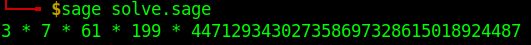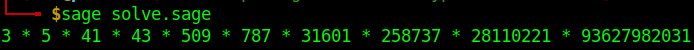Google CTF 2020 - Chunk Norris - LostMyPlaintext

#### Writeup for the easy crypto challenge from Google CTF 2020### For this challenge we get the following files:

challenge.py

```#!/usr/bin/python3 -u

import random
from Crypto.Util.number import *
import gmpy2

a = 0xe64a5f84e2762be5
chunk_size = 64

def gen_prime(bits):
s = random.getrandbits(chunk_size)

while True:
s |= 0xc000000000000001
p = 0
for _ in range(bits // chunk_size):
p = (p << chunk_size) + s
s = a * s % 2**chunk_size
if gmpy2.is_prime(p):
return p

n = gen_prime(1024) * gen_prime(1024)
e = 65537
flag = open("flag.txt", "rb").read()
print('n =', hex(n))
print('e =', hex(e))
print('c =', hex(pow(bytes_to_long(flag), e, n)))
```

output.txt

```n = 0xab802dca026b18251449baece42ba2162bf1f8f5dda60da5f8baef3e5dd49d155c1701a21c2bd5dfee142fd3a240f429878c8d4402f5c4c7f4bc630c74a4d263db3674669a18c9a7f5018c2f32cb4732acf448c95de86fcd6f312287cebff378125f12458932722ca2f1a891f319ec672da65ea03d0e74e7b601a04435598e2994423362ec605ef5968456970cb367f6b6e55f9d713d82f89aca0b633e7643ddb0ec263dc29f0946cfc28ccbf8e65c2da1b67b18a3fbc8cee3305a25841dfa31990f9aab219c85a2149e51dff2ab7e0989a50d988ca9ccdce34892eb27686fa985f96061620e6902e42bdd00d2768b14a9eb39b3feee51e80273d3d4255f6b19
e = 0x10001
```

From the second file and the last lines of "challenge.py" we can easily tell this is an RSA challenge. As for the rest of challenge.py we only get a "get_prime" function to work with heavily indicating that this is a faulty prime generator that we'll have to break. This function uses a Linear Congruential Generator (or LCG) to generate multiple 64 bit chunks that are seemingly random and then concatenates them until it produces a 1024 bit prime number p. The problem with this approach is that the randomness of each chunk is entirely dependent on the one random value the code generates, stored in the variable s. This means that if we recover s we can recover p. Which we can do with a little bit of math.

We know that in RSA the value n is equal to p*q where p and q are two primes (in this case the two primes being generated by the "gen_prime" function) so let's write p and q (using the information given to us) the following way:

• p = s1 * 264*15 + s1*a mod 264 * 264*14 + s1*a2 mod 264 * 264*13 + ... + s1*a14 mod 264 * 264*1 + s1*a15 mod 264
• q = s2 * 264*15 + s2*a mod 264 * 264*14 + s2*a2 mod 264 * 264*13 + ... + s2*a14 mod 264 * 264*1 + s2*a15 mod 264
• Where s1 and s2 refer to the two different values of s for the two different times the function "get_prime" is called and a is just the number 0xe64a5f84e2762be5 that we get from the code.

Meaning that we can write n as:

• n = s1*s2*264*30 + s1*s2*a2 mod 264 *264*29 + ... + s1*s2*a30 mod 264

Because of the line "s |= 0xc000000000000001" (which sets the first two bits as well as the last bit of s to 1) we can be sure that s1*s2 has a bit length of 128 bits. Knowing this, looking at how we've written n we can conclude that the upper 64 bits of s1*s2 are the same as the 64 upper bits of n minus a potential carry. Now that we have a way to get the 64 upper bits of s1*s2 let's figure out how to get the 64 lower bits.

Again, looking at how we've written n we can see that the lower 64 bits of n (or n mod 264) are equal to s1*s2*a30 mod 264 so we can conclude the following:

• We know that:
• s1*s2*a30 mod 264 = n mod 264
• If we multiply both sides by the Inverse Modulo of a30 modulo 264 we get:
• s1*s2 mod 264 = n*inverse_modulo(a30,264) mod 264
• (We know this inverse modulo must exist because gcd(a30,264) = 1)
• Meaning we can get the lower 64 bits of s1*s2 by calculating n*inverse_modulo(a30,264) mod 264

Now that we have both the upper 64 bits and the lower 64 bits of s1*s2 we can concatenate them to get s1*s2 and since this is a number with a bit length of 128 bits we can easily factor it(I used sagemath for this).

First I assumed there was no carry and got the following results:This doesn't seem to be correct. Remember s1 and s2 are random numbers and as such not necessarily prime so it's to be expected that s1*s2 will have multiple factors but also remember that s1 and s2 have a bit length of 64 bits. And with the numbers above it's seems impossible to get even one number with a bit length of 64 by multiplying any given combination of these numbers.

When assuming there is a carry we get the following results:Which look far more promosing.

Since we have 10 prime factors of s1*s2 what we can do is take every possible combination of these 10 numbers, run each possible combination through the following altered version of the "get_prime" function...

```def gen_prime(s):
s |= 0xc000000000000001
p = 0
for _ in range(1024//64):
p = (p << 64) + s
s = a * s % 2**64
if is_prime(p):
return p
else:
return 0
```

... and check if any result different from 0 is a factor of n. If it is we just have to divide n by it and we'll get the other one (because again n is the product of two primes). Having the factors of n all we need to do to get the flag is the usual RSA math:

• Calculate phi(n) = (p-1)*(q-1)
• (where p and q are factors of n)
• Calculate d = inverse_modulo(e,phi(n))
• And finally calculate flag = cd mod n

Full solver(written in sage):

```import binascii
import sys

n = 0xab802dca026b18251449baece42ba2162bf1f8f5dda60da5f8baef3e5dd49d155c1701a21c2bd5dfee142fd3a240f429878c8d4402f5c4c7f4bc630c74a4d263db3674669a18c9a7f5018c2f32cb4732acf448c95de86fcd6f312287cebff378125f12458932722ca2f1a891f319ec672da65ea03d0e74e7b601a04435598e2994423362ec605ef5968456970cb367f6b6e55f9d713d82f89aca0b633e7643ddb0ec263dc29f0946cfc28ccbf8e65c2da1b67b18a3fbc8cee3305a25841dfa31990f9aab219c85a2149e51dff2ab7e0989a50d988ca9ccdce34892eb27686fa985f96061620e6902e42bdd00d2768b14a9eb39b3feee51e80273d3d4255f6b19
e = 65537

a = 0xe64a5f84e2762be5

#Assuming no carry
#upperBits = bin(n)[2:][0:64]

#Assuming carry
upperBits = bin(int(bin(n)[2:][0:64],2) - 1)[2:]

assert gcd(a**30,2**64) == 1

lowerBits = bin((n*inverse_mod(a^30,2**64))%(2**64))[2:].rjust(64,'0')

assert len(upperBits) == len(lowerBits) == 64

s1s2 = int(upperBits + lowerBits, 2)

factorList = (list(factor(s1s2)))

def gen_prime(s):
s |= 0xc000000000000001
p = 0
for _ in range(1024//64):
p = (p << 64) + s
s = a * s % 2**64
if is_prime(p):
return p
else:
return 0

for num in range(3,8):
comb = Combinations(10,num).list()
for i in range(len(comb)):
s = 1
for j in range(num):
s *= factorList[comb[i][j]]
p = gen_prime(s)
if p != 0 and n%p == 0:
q = n//p
phin = (p-1)*(q-1)
d = inverse_mod(e,phin)
pt = pow(c,d,n)
print (binascii.unhexlify(hex(pt)[2:].encode()).decode())
sys.exit()
```

Flag: CTF{__donald_knuths_lcg_would_be_better_well_i_dont_think_s0__}